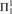Hostname: page-component-5db6c4db9b-5lzww Total loading time: 0 Render date: 2023-03-26T13:35:23.559Z Has data issue: true Feature Flags: { "useRatesEcommerce": false } hasContentIssue true

# A NEW COMPUTATION OF THE Σ-ORDINAL OF KPω

Published online by Cambridge University Press:  17 April 2014

## Abstract

We define a functional interpretation of KPω using Howard’s primitive recursive tree functionals of finite type and associated terms. We prove that the Σ-ordinal of KPω is the least ordinal not given by a closed term of the ground type of the trees (the Bachmann-Howard ordinal). We also extend KPω to a second-order theory with Δ1-comprehension and strict-${\rm{\Pi }}_1^1$ reflection and show that the Σ-ordinal of this theory is still the Bachmann-Howard ordinal. It is also argued that the second-order theory is Σ1-conservative over KPω.

Type
Articles
Information
The Journal of Symbolic Logic , March 2014 , pp. 306 - 324

## Access options

Get access to the full version of this content by using one of the access options below. (Log in options will check for institutional or personal access. Content may require purchase if you do not have access.)

## References

#### REFERENCES

Avigad, J. and Feferman, S., Gödel’s functional (“Dialectica”) interpretation, Handbook of proof theory (Buss, S. R., editor), Studies in Logic and the Foundations of Mathematics, vol. 137, North Holland, Amsterdam, 1998, pp. 337405.Google Scholar
Avigad, J. and Towsner, H., Functional interpretation and inductive definitions, this Journal, vol. 74 (2009), no. 4, pp. 11001120.Google Scholar
Bachmann, H., Die Normalfunktionen und das Problem der ausgezeichneten Folgen von Ordnungszahlen. Vierteljahrsschrift der Naturforschenden Gesellschaft in Zürich, vol. 95 (1950), pp. 115–147.
Barwise, J., Admissible set theory and structures: An approach to definability theory, Perspectives in Mathematical Logic, Springer-Verlag, Berlin, 1975.CrossRefGoogle Scholar
Burr, W., A Diller-Nahm-style functional interpretation of KPω. Archive for Mathematical Logic, vol. 39 (2000), pp. 599604.Google Scholar
Burr, W. and Hartung, V., A characterization of theΣ-definable functions of KPW + (uniform ) AC. Archive for Mathematical Logic, vol. 37 (1998), no. 3, pp. 199–214.
Diller, J. and Nahm, W., Eine Variante zur Dialectica-Interpretation der Heyting-Arithmetik endlicher Typen. Archive für mathematische Logik und Grundlagenforschung, vol. 16 (1974), pp. 4966.Google Scholar
Feferman, S., The proof theory of classical and constructive inductive definitions. A 40-year saga, 1968–2008, Ways of proof theory (Ralph Schindler, editor), Ontos Mathematical Logic, vol. 2, Ontos Verlag, Frankfurt, 2011, pp. 7–29.
Ferreira, F., Injecting uniformities into Peano arithmetic. Annals of Pure and Applied Logic, vol. 157 (2009), pp. 122129.Google Scholar
Ferreira, F. and Ferreira, G., Harrington’s conservation theorem redone. Archive for Mathematical Logic, vol. 47 (2008), no. 2, pp. 91100.Google Scholar
Ferreira, F. and Oliva, P., Bounded functional interpretation. Annals of Pure and Applied Logic, vol. 135 (2005), pp. 73112.Google Scholar
Ferreira, F. and Oliva, P., On bounded functional interpretations. Annals of Pure and Applied Logic, vol. 163 (2012), no. 8, pp. 10301049.Google Scholar
Gödel, K., Über eine bisher noch nicht benützte Erweiterung des finiten Standpunktes. dialectica, vol. 12 (1958), pp. 280–287, Reprinted with an English translation in 15, pp. 240251.Google Scholar
Gödel, K., On an extension of finitary mathematics which has not yet been used, 1972, Published in 15, pages 271–280. Revised version of 13.
Gödel, K., Collected works , (Feferman et al., editors), vol. II, Oxford University Press, Oxford, 1990.
Howard, W. A., A system of abstract constructive ordinals, this Journal, vol. 37 (1972), no. 2, pp. 355–374.
Jäger, G., Die konstruktible Hierarchie als Hilfsmittel zur beweistheoretischen Untersuchung von Teilsystemen der Mengenlehre und Analysis , Ph.D. thesis, Ludwig-Maximilians-Universität, Munich, 1979.
Oliva, P., Unifying functional interpretations. Notre Dame Journal of Formal Logic, vol. 47 (2006), pp. 263290.Google Scholar
Pohlers, W., Proof theory. The first step into impredicativity , Universitext, Springer-Verlag, Berlin, 2009.
Sacks, G., Higher recursion theory , Perspectives in Mathematical Logic, Springer-Verlag, Berlin, 1990.
Salipante, V., On the consistency strenght of the strict Π1 reflection principle, Ph.D. thesis, Universität Bern, 2005.
Shoenfield, J. R., Mathematical logic , Addison-Wesley Publishing Company, 1967, Republished in 2001 by AK Peters.
Simpson, S. G., Subsystems of second order arithmetic , Perspectives in Mathematical Logic, Springer, Berlin, 1999.
Stein, M., Interpretationen der Heyting-Arithmetik endlicher Typen. Archive für Mathematische Logik und Grundlagenforschung, vol. 19 (1979), pp. 4966.Google Scholar
Troelstra, A. S., Introductory note to 13 and 14, 1990, Published in 15, pp. 214–241.
Troelstra, A. S., Metamathematical investigation of intuitionistic arithmetic and analysis, Lecture Notes in Mathematics, vol. 344, Springer, Berlin, 1973.CrossRefGoogle Scholar